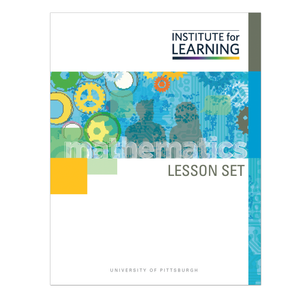# Exploring Multiplicative Comparisons (Grade 4)

Regular price \$6.25 \$0.00 Unit price per

This lesson set provides a study of multiplicative comparisons, problems in which students have to solve for “how many times greater/less.” Students will:

• explore the meaning of the two factors in comparison multiplication problems;
• recognize the inverse relationship between multiplication and division, and determine that division can be used to solve comparison multiplication problems when either the group size or the scaling factor is provided; and
• use a variety of representations to respond to a problem situation, and weigh the advantages and disadvantages of such representations.

Standards: 4.OA.A.1, 4.OA.A.2